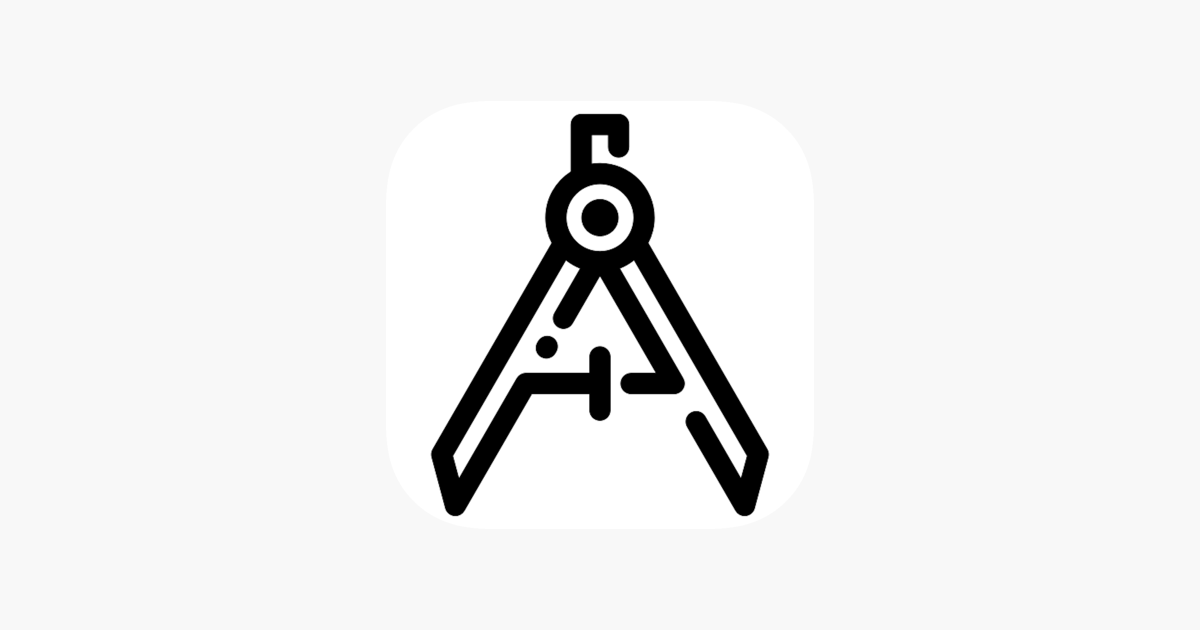Arithmatic Subject – Frank CarterAs the name implies Arithmetic Subject it contain some symbol which is used to represent the arithmetic operations.

This application contain addition, subtraction, multiplication and division etc.

With the help of numbers which is displayed you can easily perform the task with the given options, and this are the basic arithmetic operators.

The Arithmetic Subject contain two values which is used for addition, subtraction, multiplication and division.

Features:

-Easy to learn the operation.

-it is used to perform the sequential calculation.

-All the number operator contain real number to perform any task.

-there are four category different such as addition, subtraction, multiplication

and division and choose any of them.

How to Use:

-Choose the any category.

-Identify number values.

-Calculate the number.